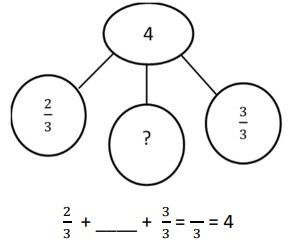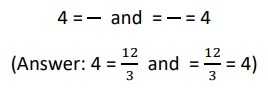# 3rd Grade, Activity 270: Develop Understanding of Fractions as Numbers

Explain equivalence of fractions with denominators 2, 3, 4, 6, and 8 in special cases, and compare fractions by reasoning about their size.

c. Express whole numbers as fractions, and recognize fractions that are equivalent to whole numbers. Examples: Express 3 in the form 3 = 3/1; recognize that 6/1 = 6; locate 4/4 and 1 at the same point of a number line diagram.

### Number Bond Fractions

• Group: Whole Class/Alone
• Materials: paper and pencil, Number Bond Fractions sheet

Draw a diagram like the one shown below on the board. Have students draw a similar diagram on their papers.
Explain that number bonds help students recognize fractions that are equivalent to the identified whole numbers.To determine the fraction that is equal to the whole number, pose the questions below:

• What does the denominator tell about the divisions of the fractions? (The number of total parts.)
• How many thirds are in 0? (0 thirds)
• How many thirds are in 1? (3 thirds)
• How many thirds are in 2? (6 thirds)
• How many thirds are in 3? (9 thirds)
• How many thirds are in 4? (12 thirds)
• How many thirds can be added to two-thirds and three-thirds to get 12 thirds? (7 thirds)

Draw the models like the ones below on the board. Have students place the numbers appropriately in the models
below. Direct students to draw the models on their papers.Provide other examples.

Issue a Number Bond Fraction sheet to each student. Instruct students to complete the number bonds to find the missing fraction whose total is equivalent to the whole number in the number bonds. Then have them use the number bonds to express whole numbers as fractions and vice versa. Assign volunteers problems to complete at the board so the rest of the students can compare their answers to those modeled on the board.